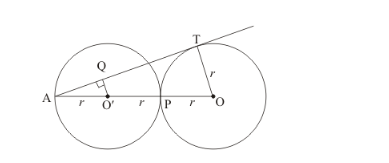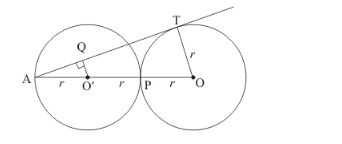# Two circles of same radii r and centres O and O' touch each other at P as shown in Fig.

Question:

Two circles of same radii r and centres O and O' touch each other at P as shown in Fig. 10.91. If O O' is produced to meet the cirele C (O', r) at A and AT is a tangent to the circle C (O,r) such that O'Q "> AT. Then AO : AO' =(a) 3/2

(b) 2

(c) 3

(d) 1/4

Solution:

From the given figure we have,AO = r + r + r

AO = 3r

AO’ = r

Therefore,

$\frac{A O}{A O^{\prime}}=\frac{3 r}{r}$

$\frac{A O}{A O^{\prime}}=3$

Also as $O^{\prime} Q \| O T$ therefore $\frac{A T}{A Q}=\frac{A O}{A O^{\prime}}$

Therefore, option (c) is correct.